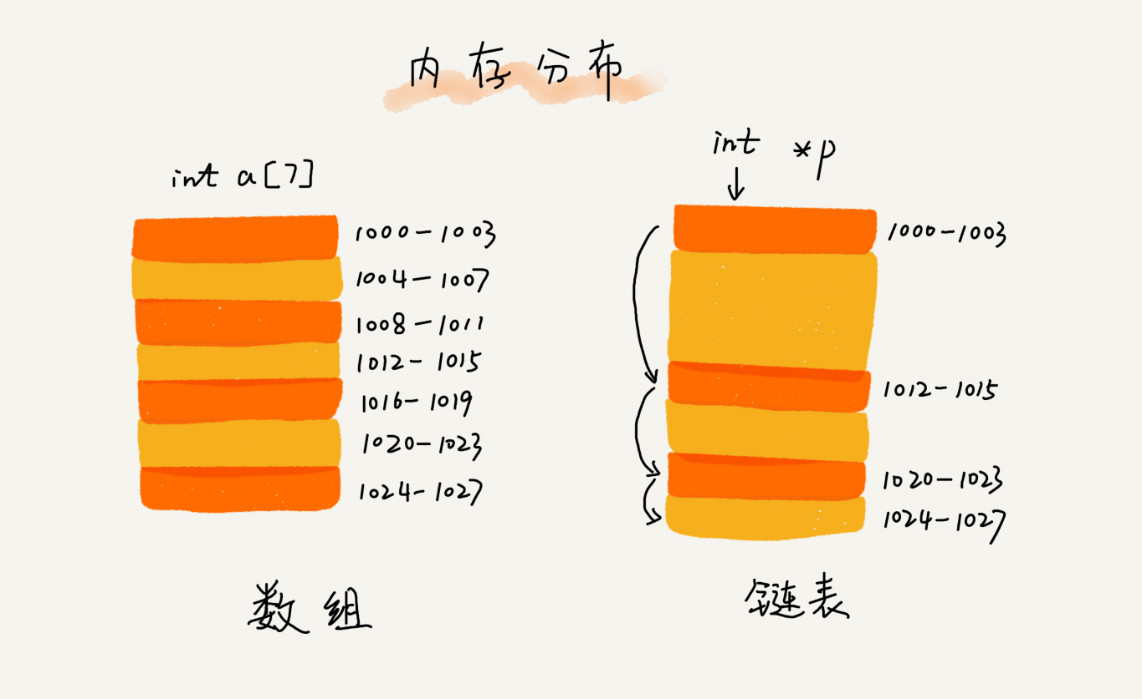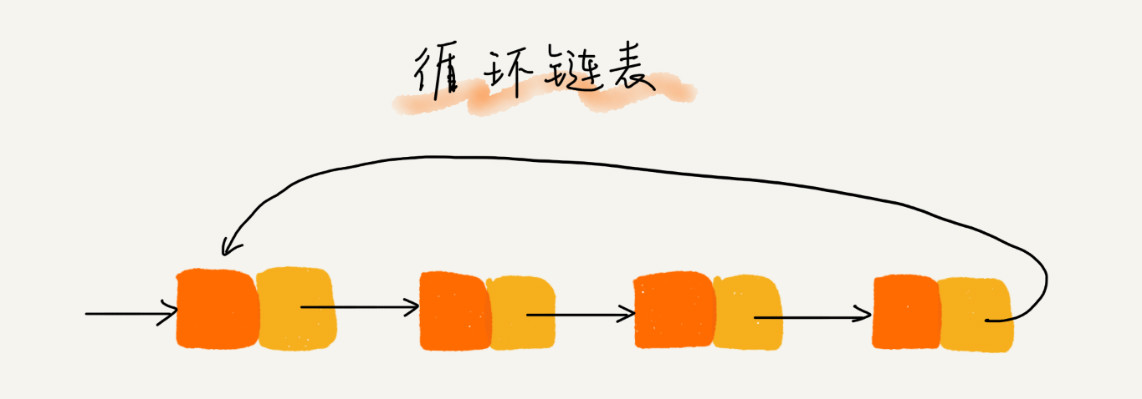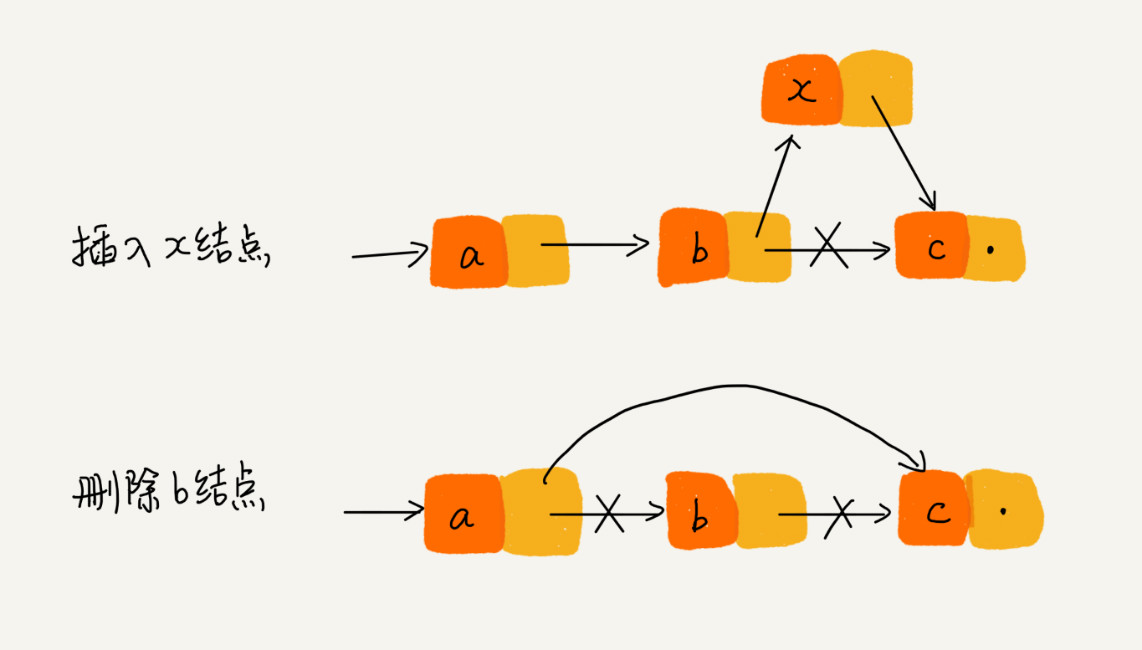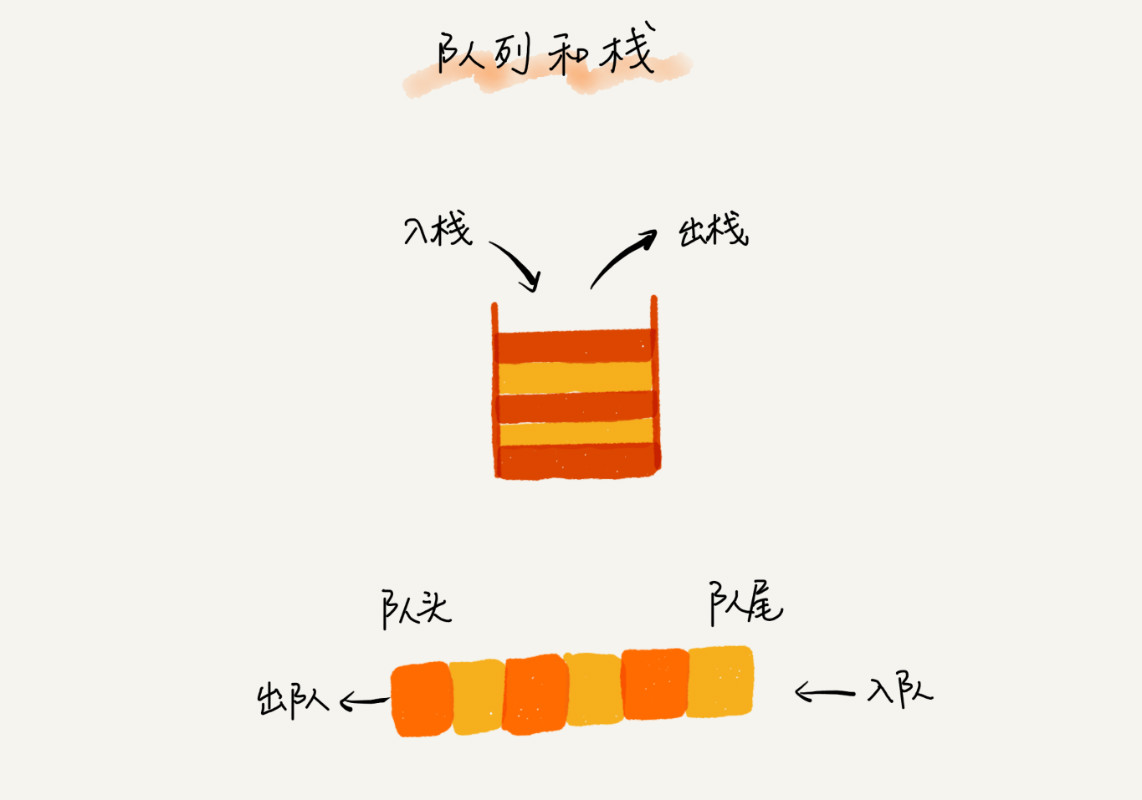Java

# 一、数据结构通讲

## 1.链表

### ①链表基本介绍（图片来自王争的数据结构与算法之美）（图片来自王争的数据结构与算法之美）（来自王争的数据结构与算法之美）

## 3.队列（来自王争的数据结构与算法之美）### 2.1内部类Node

``````private static class Node<E> {
//值
E item;
//下一个结点的指针
Node<E> next;
//上一个结点的指针
Node<E> prev;

Node(Node<E> prev, E element, Node<E> next) {
this.item = element;
this.next = next;
this.prev = prev;
}
}
``````

### 2.2属性

``````	//当前list中元素的数量
transient int size = 0;
/**
* 指向第一个结点的指针
*/
transient Node<E> first;

/**
* 指向最后一个结点的指针
*/
transient Node<E> last;
``````

### 2.3 构造方法

``````public LinkedList() {
}

public LinkedList(Collection<? extends E> c) {
this();
}
``````

PS：这里并没有像ArrayList那样有规定初始大小的构造函数，因为链表容量本来就是可以增加的，所以不需要有这样的构造函数。

``````public boolean addAll(Collection<? extends E> c) {
}
``````
``````public boolean addAll(int index, Collection<? extends E> c) {
checkPositionIndex(index);
//调用集合c的toArray方法将集合类对象转化为对象数组
Object[] a = c.toArray();
int numNew = a.length;
if (numNew == 0)
return false;

Node<E> pred, succ;
//找到对应下标的结点
if (index == size) {
succ = null;
pred = last;
} else {
succ = node(index);
pred = succ.prev;
}
//循环插入
for (Object o : a) {
@SuppressWarnings("unchecked")
E e = (E) o;
Node<E> newNode = new Node<>(pred, e, null);
if (pred == null)
first = newNode;
else
pred.next = newNode;
pred = newNode;
}
//最后一个结点的处理
if (succ == null) {
last = pred;
} else {
pred.next = succ;
succ.prev = pred;
}

size += numNew;
//修改操作计数+1
modCount++;
return true;
}
``````

## 3.List接口的常用方法

``````public boolean add(E e) {
return true;
}
``````

``````//向尾部增加一个元素
//最后一个结点
final Node<E> l = last;
//创建一个新的结点对象
final Node<E> newNode = new Node<>(l, e, null);
//更新last指针为新的
last = newNode;
if (l == null)
first = newNode;
else
l.next = newNode;
//元素数量自增1
size++;
//修改的数量
modCount++;
}
``````

### ②remove（Object o）

``````//遍历所有结点，如果是该对象则删除结点，返回true;否则返回false表示未找到
public boolean remove(Object o) {
if (o == null) {
for (Node<E> x = first; x != null; x = x.next) {
if (x.item == null) {
return true;
}
}
} else {
for (Node<E> x = first; x != null; x = x.next) {
if (o.equals(x.item)) {
return true;
}
}
}
return false;
}
``````

``````E unlink(Node<E> x) {
// assert x != null;
final E element = x.item;
final Node<E> next = x.next;
final Node<E> prev = x.prev;

//判断是不是首结点
if (prev == null) {
//如果是则first指针指向下一个即可
first = next;
} else {
//如果不是则将前一个结点指向后一个结点
prev.next = next;
x.prev = null;
}
//判断是不是尾结点
if (next == null) {
last = prev;
} else {
next.prev = prev;
x.next = null;
}
//将其值改为null,其实按理来说只需指针丢弃即可在逻辑上将结点从链表上删除，但这里可能是想让拥有这个结点指针的程序知道这个结点已经被删除了，同时把结点的值对象指针丢弃也利于gc回收
x.item = null;
//更新size
size--;
//修改计数+1
modCount++;
return element;
}
``````

### ③remove(int index)

``````public E remove(int index) {
//边界检查，就是看看index合不合法
checkElementIndex(index);
}
``````

``````Node<E> node(int index) {
//把index下标与size的一半去比较，言外之意就是比较index是在前半部分还是后半部分，这样可以较快效率
if (index < (size >> 1)) {
//如果在前半部分便从头结点开始遍历
Node<E> x = first;
for (int i = 0; i < index; i++)
x = x.next;
return x;
} else {
//如果在后半部分则从后半部分开始遍历
Node<E> x = last;
for (int i = size - 1; i > index; i--)
x = x.prev;
return x;
}
}
``````

### ④set(int index, E element)

``````public E set(int index, E element) {
//下标检查
checkElementIndex(index);
//查找对应的结点
Node<E> x = node(index);
E oldVal = x.item;
//更新值
x.item = element;
//返回旧值
return oldVal;
}
``````

### ⑤indexOf(Object o)

``````public int indexOf(Object o) {
int index = 0;
if (o == null) {
for (Node<E> x = first; x != null; x = x.next) {
if (x.item == null)
return index;
index++;
}
} else {
for (Node<E> x = first; x != null; x = x.next) {
if (o.equals(x.item))
return index;
index++;
}
}
return -1;
}
``````

### ⑥lastIndexOf(Object o)

``````public int lastIndexOf(Object o) {
int index = size;
if (o == null) {
for (Node<E> x = last; x != null; x = x.prev) {
index--;
if (x.item == null)
return index;
}
} else {
for (Node<E> x = last; x != null; x = x.prev) {
index--;
if (o.equals(x.item))
return index;
}
}
return -1;
}
``````

``````public void clear() {
// Clearing all of the links between nodes is "unnecessary", but:
// - helps a generational GC if the discarded nodes inhabit
//   more than one generation
// - is sure to free memory even if there is a reachable Iterator
for (Node<E> x = first; x != null; ) {
Node<E> next = x.next;
x.item = null;
x.next = null;
x.prev = null;
x = next;
}
first = last = null;
size = 0;
modCount++;
}
``````

## 4.Queue接口的常用方法

``````Queue<Integer> queue=new LinkedList();
//入队
//打印队首元素
System.out.println(queue.peek());
//出队
queue.poll();
``````

``````public boolean add(E e) {
//创建一个值为e的结点，加到队尾
return true;
}
``````

### ②poll()

``````public E poll() {
final Node<E> f = first;
return (f == null) ? null : unlinkFirst(f);
}
``````

### ③peek()

``````public E peek() {
final Node<E> f = first;
return (f == null) ? null : f.item;
}
``````

## 5.Deque接口的常用方法

Deque是个双向队列的接口，但实际上也是可以用来当做栈使用的。就像下面这样，

``````Deque<Integer> stack=new LinkedList();
//入栈
stack.push(1);
//输出栈顶元素
System.out.println(stack.peek());
//出栈
stack.pop();
``````

### ①push(E e)

``````public void push(E e) {
}
``````

### ②pop()

``````public E pop() {
return removeFirst();
}
``````

### ①ListItr

``````private class ListItr implements ListIterator<E> {
private Node<E> lastReturned;
private Node<E> next;
private int nextIndex;
private int expectedModCount = modCount;

ListItr(int index) {
// assert isPositionIndex(index);
next = (index == size) ? null : node(index);
nextIndex = index;
}

public boolean hasNext() {
return nextIndex < size;
}

public E next() {
//检查是否存在预期外的修改
checkForComodification();
//判断next是否越界
if (!hasNext())
throw new NoSuchElementException();
//指针后移
lastReturned = next;
next = next.next;
//下标+1
nextIndex++;
return lastReturned.item;
}

public boolean hasPrevious() {
return nextIndex > 0;
}

public E previous() {
checkForComodification();
if (!hasPrevious())
throw new NoSuchElementException();

lastReturned = next = (next == null) ? last : next.prev;
nextIndex--;
return lastReturned.item;
}

public int nextIndex() {
return nextIndex;
}

public int previousIndex() {
return nextIndex - 1;
}

public void remove() {
checkForComodification();
if (lastReturned == null)
throw new IllegalStateException();

Node<E> lastNext = lastReturned.next;
if (next == lastReturned)
next = lastNext;
else
nextIndex--;
lastReturned = null;
expectedModCount++;
}

public void set(E e) {
if (lastReturned == null)
throw new IllegalStateException();
checkForComodification();
lastReturned.item = e;
}

checkForComodification();
lastReturned = null;
if (next == null)
else
nextIndex++;
//预期修改增加
expectedModCount++;
}

public void forEachRemaining(Consumer<? super E> action) {
//检查action参数对象是否为null
Objects.requireNonNull(action);
//遍历执行action的accept方法，把结点的值作为参数传入
while (modCount == expectedModCount && nextIndex < size) {
action.accept(next.item);
lastReturned = next;
next = next.next;
nextIndex++;
}
//检查是否有预期外的修改
checkForComodification();
}

final void checkForComodification() {
if (modCount != expectedModCount)
throw new ConcurrentModificationException();
}
}
``````

### ②DescendingIterator 逆序迭代器

``````private class DescendingIterator implements Iterator<E> {
private final ListItr itr = new ListItr(size());
public boolean hasNext() {
return itr.hasPrevious();
}
public E next() {
return itr.previous();
}
public void remove() {
itr.remove();
}
}
``````

### ③DescendingIterator 拆分迭代器

``````static final class LLSpliterator<E> implements Spliterator<E> {
static final int BATCH_UNIT = 1 << 10;  // batch array size increment
static final int MAX_BATCH = 1 << 25;  // max batch array size;
final LinkedList<E> list; // null OK unless traversed
Node<E> current;      // current node; null until initialized
int est;              // size estimate; -1 until first needed
int expectedModCount; // initialized when est set
int batch;            // batch size for splits

LLSpliterator(LinkedList<E> list, int est, int expectedModCount) {
this.list = list;
this.est = est;
this.expectedModCount = expectedModCount;
}

final int getEst() {
int s; // force initialization
if ((s = est) < 0) {
if ((lst = list) == null)
s = est = 0;
else {
expectedModCount = lst.modCount;
current = lst.first;
s = est = lst.size;
}
}
return s;
}

public long estimateSize() { return (long) getEst(); }

public Spliterator<E> trySplit() {
Node<E> p;
int s = getEst();
if (s > 1 && (p = current) != null) {
int n = batch + BATCH_UNIT;
if (n > s)
n = s;
if (n > MAX_BATCH)
n = MAX_BATCH;
Object[] a = new Object[n];
int j = 0;
do { a[j++] = p.item; } while ((p = p.next) != null && j < n);
current = p;
batch = j;
est = s - j;
return Spliterators.spliterator(a, 0, j, Spliterator.ORDERED);
}
return null;
}

public void forEachRemaining(Consumer<? super E> action) {
Node<E> p; int n;
if (action == null) throw new NullPointerException();
if ((n = getEst()) > 0 && (p = current) != null) {
current = null;
est = 0;
do {
E e = p.item;
p = p.next;
action.accept(e);
} while (p != null && --n > 0);
}
if (list.modCount != expectedModCount)
throw new ConcurrentModificationException();
}

public boolean tryAdvance(Consumer<? super E> action) {
Node<E> p;
if (action == null) throw new NullPointerException();
if (getEst() > 0 && (p = current) != null) {
--est;
E e = p.item;
current = p.next;
action.accept(e);
if (list.modCount != expectedModCount)
throw new ConcurrentModificationException();
return true;
}
return false;
}

public int characteristics() {
return Spliterator.ORDERED | Spliterator.SIZED | Spliterator.SUBSIZED;
}
}
``````

# 三、总结

1. LinedList实际是由链表实现，是一种双向链表的实现。
•